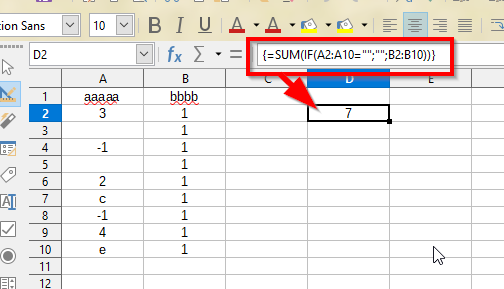# SUMIF with different Criteria values?

I’m attempting to use the SUMIF function to sum the total of numbers in column B based on the values in column A. The values in column A could be either numbers or letters. If a cell is not blank in column A I’m looking for the corrosponding number in column B to be summed.

Tried using wildcards as the Criteria in the SUMIF formula but to no avail. Also tried playing with ISBLANK=0, couldnt get that to work either.

Any thoughts?

A direct answer without using array formula:

``````=SUMIF(A1:A10;"<>";B1:B10)
``````

Rationale: the “search criterion” in the SUMIF is defined in OASIS ODF specification:

For `<>`, if the value is empty it matches non-empty cells.

filed tdf#131995

Not relevant, but I prefer to use always SUMIFS() because the SumRange it’s at the beginning, and it’s easy to add new conditions if it is the case.

Thank you Mike, this works and is exactly what I was looking for.

Enter the formula, `=SUM(IF(A2:A10="";"";B2:B10))` and use Ctrl+Shift+Enter, it’s matrix formula.ATTENTION: If you would like to give more details to your question, use edit in question or add a comment below. Thank you.

If the answer met your need, please click on the ballto the left of the answer, to finish the question.

Thank you Schiavinatto, appreciate the response. My actual situation is a bit more complicated than my simplified example above, and I couldn’t get this to easily work for me. Mikes answer worked perfectly for my particular sheet setup.